# 神经网络轻松制作

16 三月 2020, 11:56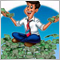0
2 522

### 1. AI 网络建设原理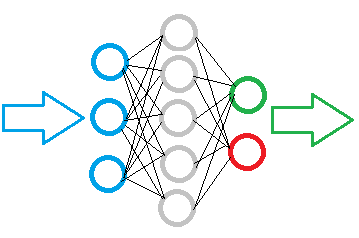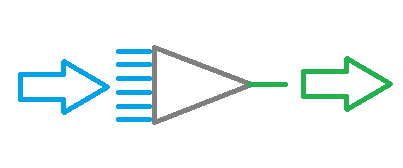• 一个神经网络是由相同的神经元构成的，因此，一个种类的神经元就足以建立一个模型。
• 模型中的神经元是分层组织的；
• 神经网络中的数据流在实现时，于模型的所有层之间从输入神经元串行传输数据到输出神经元。
• 输入神经元的数量取决于每一遍所分析的数据量，而输出神经元的数量取决于结果数据量；
• 由于逻辑结果会在输出端形成，因此为神经网络提出的问题应能得到无歧义的答案。

### 2. 人工神经元的结构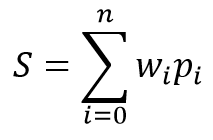• Sigmoid 函数 — 返回值的范围从 “0” 到 “1”
• 双曲正切 — 返回值的范围从 “-1” 到 “1”

• 监督学习；
• 无监督学习；
• 强化学习。

### 4. 利用 MQL 构建我们自己的神经网络

#### 4.1. 连接

```class СConnection : public CObject
{
public:
double            weight;
double            deltaWeight;
СConnection(double w) { weight=w; deltaWeight=0; }
~СConnection(){};
//--- methods for working with files
virtual bool      Save(const int file_handle);
};

```

```bool СConnection::Save(const int file_handle)
{
if(file_handle==INVALID_HANDLE)
return false;
//---
if(FileWriteDouble(file_handle,weight)<=0)
return false;
if(FileWriteDouble(file_handle,deltaWeight)<=0)
return false;
//---
return true;
}

```

```class CArrayCon  :    public CArrayObj
{
public:
CArrayCon(void){};
~CArrayCon(void){};
//---
virtual bool      CreateElement(const int index);
virtual int       Type(void) const { return(0x7781); }
};

```

```bool CArrayCon::CreateElement(const int index)
{
if(index<0)
return false;
//---
if(m_data_max<index+1)
{
if(ArrayResize(m_data,index+10)<=0)
return false;
m_data_max=ArraySize(m_data)-1;
}
//---
m_data[index]=new СConnection(MathRand()/32767.0);
if(!CheckPointer(m_data[index])!=POINTER_INVALID)
return false;
m_data_total=MathMax(m_data_total,index);
//---
return (true);
}

```

#### 4.2. 神经元

```class CNeuron  :  public CObject
{
public:
CNeuron(uint numOutputs,uint myIndex);
~CNeuron() {};
void              setOutputVal(double val) { outputVal=val; }
double            getOutputVal() const { return outputVal; }
void              feedForward(const CArrayObj *&prevLayer);
void              updateInputWeights(CArrayObj *&prevLayer);
//--- methods for working with files
virtual bool      Save(const int file_handle)                         { return(outputWeights.Save(file_handle));   }

private:
double            eta;
double            alpha;
static double     activationFunction(double x);
static double     activationFunctionDerivative(double x);
double            sumDOW(const CArrayObj *&nextLayer) const;
double            outputVal;
CArrayCon         outputWeights;
uint              m_myIndex;
};

```

```CNeuron::CNeuron(uint numOutputs, uint myIndex)  :  eta(0.15), // net learning rate
alpha(0.5) // momentum
{
for(uint c=0; c<numOutputs; c++)
{
outputWeights.CreateElement(c);
}

m_myIndex=myIndex;
}

```

setOutputVal 和 getOutputVal 方法用于访问神经元的结果值。 神经元的结果值是在 feedForward 方法中计算的。 前一层神经元作为该方法的输入参数。

```void CNeuron::feedForward(const CArrayObj *&prevLayer)
{
double sum=0.0;
int total=prevLayer.Total();
for(int n=0; n<total && !IsStopped(); n++)
{
CNeuron *temp=prevLayer.At(n);
double val=temp.getOutputVal();
if(val!=0)
{
СConnection *con=temp.outputWeights.At(m_myIndex);
sum+=val * con.weight;
}
}
outputVal=activationFunction(sum);
}

```

```double CNeuron::activationFunction(double x)
{
//output range [-1.0..1.0]
return tanh(x);
}

```

```double CNeuron::activationFunctionDerivative(double x)
{
return 1/MathPow(cosh(x),2);
}

```

```void CNeuron::calcHiddenGradients(const CArrayObj *&nextLayer)
{
double dow=sumDOW(nextLayer);
}
//+------------------------------------------------------------------+
//|                                                                  |
//+------------------------------------------------------------------+
{
double delta=targetVals-outputVal;
}

```

```double CNeuron::sumDOW(const CArrayObj *&nextLayer) const
{
double sum=0.0;
int total=nextLayer.Total()-1;
for(int n=0; n<total; n++)
{
СConnection *con=outputWeights.At(n);
CNeuron *neuron=nextLayer.At(n);
}
return sum;
}

```

```void CNeuron::updateInputWeights(CArrayObj *&prevLayer)
{
int total=prevLayer.Total();
for(int n=0; n<total && !IsStopped(); n++)
{
CNeuron *neuron= prevLayer.At(n);
СConnection *con=neuron.outputWeights.At(m_myIndex);
}
}

```

#### 4.3. 神经网络

```class CLayer: public CArrayObj
{
private:
uint              iOutputs;
public:
CLayer(const int outputs=0) { iOutputs=outpus; };
~CLayer(void){};
//---
virtual bool      CreateElement(const int index);
virtual int       Type(void) const { return(0x7779); }
};

```

```bool CLayer::CreateElement(const uint index)
{
if(index<0)
return false;
//---
if(m_data_max<index+1)
{
if(ArrayResize(m_data,index+10)<=0)
return false;
m_data_max=ArraySize(m_data)-1;
}
//---
CNeuron *neuron=new CNeuron(iOutputs,index);
if(!CheckPointer(neuron)!=POINTER_INVALID)
return false;
neuron.setOutputVal((neuronNum%3)-1)
//---
m_data[index]=neuron;
m_data_total=MathMax(m_data_total,index);
//---
return (true);
}

```

```class CArrayLayer  :    public CArrayObj
{
public:
CArrayLayer(void){};
~CArrayLayer(void){};
//---
virtual bool      CreateElement(const uint neurons, const uint outputs);
virtual int       Type(void) const { return(0x7780); }
};

```

```bool CArrayLayer::CreateElement(const uint neurons, const uint outputs)
{
if(neurons<=0)
return false;
//---
if(m_data_max<=m_data_total)
{
if(ArrayResize(m_data,m_data_total+10)<=0)
return false;
m_data_max=ArraySize(m_data)-1;
}
//---
CLayer *layer=new CLayer(outputs);
if(!CheckPointer(layer)!=POINTER_INVALID)
return false;
for(uint i=0; i<neurons; i++)
if(!layer.CreatElement(i))
return false;
//---
m_data[m_data_total]=layer;
m_data_total++;
//---
return (true);
}

```

```class CNet
{
public:
CNet(const CArrayInt *topology);
~CNet(){};
void              feedForward(const CArrayDouble *inputVals);
void              backProp(const CArrayDouble *targetVals);
void              getResults(CArrayDouble *&resultVals);
double            getRecentAverageError() const { return recentAverageError; }
bool              Save(const string file_name, double error, double undefine, double forecast, datetime time, bool common=true);
bool              Load(const string file_name, double &error, double &undefine, double &forecast, datetime &time, bool common=true);
//---
static double     recentAverageSmoothingFactor;
private:
CArrayLayer       layers;
double            recentAverageError;
};

```

```CNet::CNet(const CArrayInt *topology)
{
if(CheckPointer(topology)==POINTER_INVALID)
return;
//---
int numLayers=topology.Total();
for(int layerNum=0; layerNum<numLayers; layerNum++)
{
uint numOutputs=(layerNum==numLayers-1 ? 0 : topology.At(layerNum+1));
if(!layers.CreateElement(topology.At(layerNum), numOutputs))
return;
}
}

```

feedForward 方法计算神经网络值。 在参数中，该方法接收基于计算神经网络的结果值计算得出的输入值数组。

```void CNet::feedForward(const CArrayDouble *inputVals)
{
if(CheckPointer(inputVals)==POINTER_INVALID)
return;
//---
CLayer *Layer=layers.At(0);
if(CheckPointer(Layer)==POINTER_INVALID)
{
return;
}
int total=inputVals.Total();
if(total!=Layer.Total()-1)
return;
//---
for(int i=0; i<total && !IsStopped(); i++)
{
CNeuron *neuron=Layer.At(i);
neuron.setOutputVal(inputVals.At(i));
}
//---
total=layers.Total();
for(int layerNum=1; layerNum<total && !IsStopped(); layerNum++)
{
CArrayObj *prevLayer = layers.At(layerNum - 1);
CArrayObj *currLayer = layers.At(layerNum);
int t=currLayer.Total()-1;
for(int n=0; n<t && !IsStopped(); n++)
{
CNeuron *neuron=currLayer.At(n);
neuron.feedForward(prevLayer);
}
}
}

```

```void CNet::getResults(CArrayDouble *&resultVals)
{
if(CheckPointer(resultVals)==POINTER_INVALID)
{
resultVals=new CArrayDouble();
}
resultVals.Clear();
CArrayObj *Layer=layers.At(layers.Total()-1);
if(CheckPointer(Layer)==POINTER_INVALID)
{
return;
}
int total=Layer.Total()-1;
for(int n=0; n<total; n++)
{
CNeuron *neuron=Layer.At(n);
}
}

```

```void CNet::backProp(const CArrayDouble *targetVals)
{
if(CheckPointer(targetVals)==POINTER_INVALID)
return;
CArrayObj *outputLayer=layers.At(layers.Total()-1);
if(CheckPointer(outputLayer)==POINTER_INVALID)
return;
//---
double error=0.0;
int total=outputLayer.Total()-1;
for(int n=0; n<total && !IsStopped(); n++)
{
CNeuron *neuron=outputLayer.At(n);
double delta=targetVals[n]-neuron.getOutputVal();
error+=delta*delta;
}
error/= total;
error = sqrt(error);

recentAverageError+=(error-recentAverageError)/recentAverageSmoothingFactor;
//---
for(int n=0; n<total && !IsStopped(); n++)
{
CNeuron *neuron=outputLayer.At(n);
}
//---
for(int layerNum=layers.Total()-2; layerNum>0; layerNum--)
{
CArrayObj *hiddenLayer=layers.At(layerNum);
CArrayObj *nextLayer=layers.At(layerNum+1);
total=hiddenLayer.Total();
for(int n=0; n<total && !IsStopped();++n)
{
CNeuron *neuron=hiddenLayer.At(n);
}
}
//---
for(int layerNum=layers.Total()-1; layerNum>0; layerNum--)
{
CArrayObj *layer=layers.At(layerNum);
CArrayObj *prevLayer=layers.At(layerNum-1);
total=layer.Total()-1;
for(int n=0; n<total && !IsStopped(); n++)
{
CNeuron *neuron=layer.At(n);
neuron.updateInputWeights(prevLayer);
}
}
}

```

### 本文用到的程序

# 名称 类型 说明
1 NeuroNet.mqh 类库 创建神经网络（感知器）的类库

NeuroNet.mqh (38.34 KB)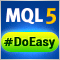轻松快捷开发 MetaTrader 程序的函数库（第 二十九部分）：延后交易请求 - 请求对象类轻松快捷开发 MetaTrader 程序的函数库（第 二十八部分）：延后交易请求之平仓、删除和修改轻松快捷开发 MetaTrader 程序的函数库（第 三十部分）：延后交易请求 - 管理请求对象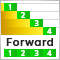连续前行优化 (第三部分): 将机器人适配为自动优化器